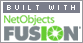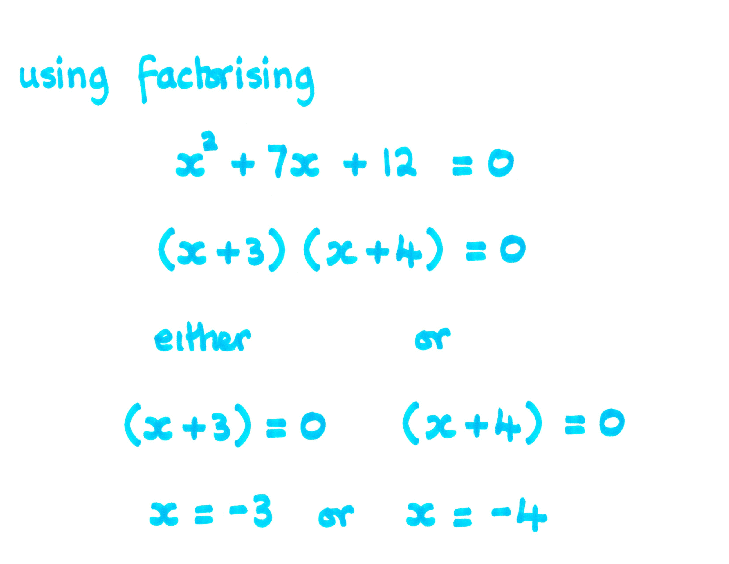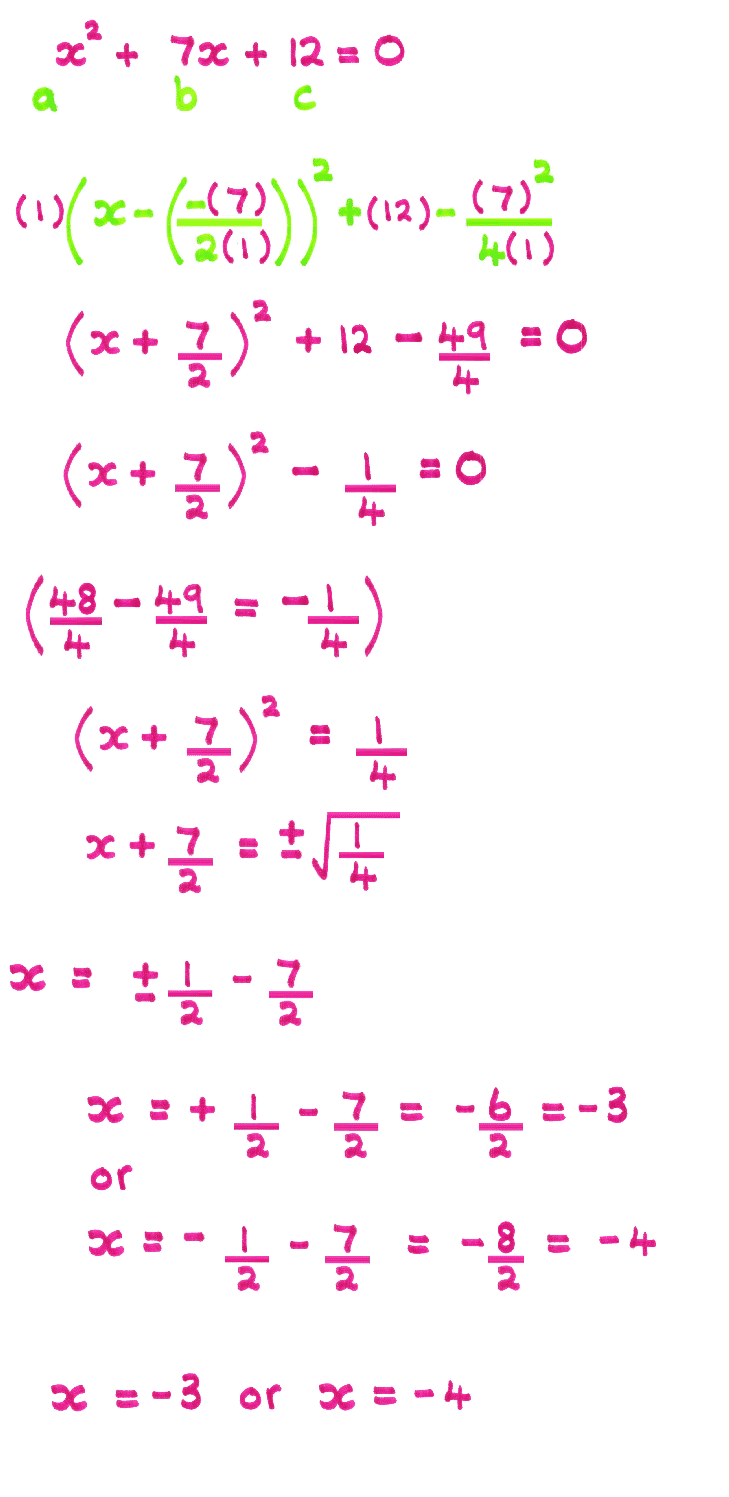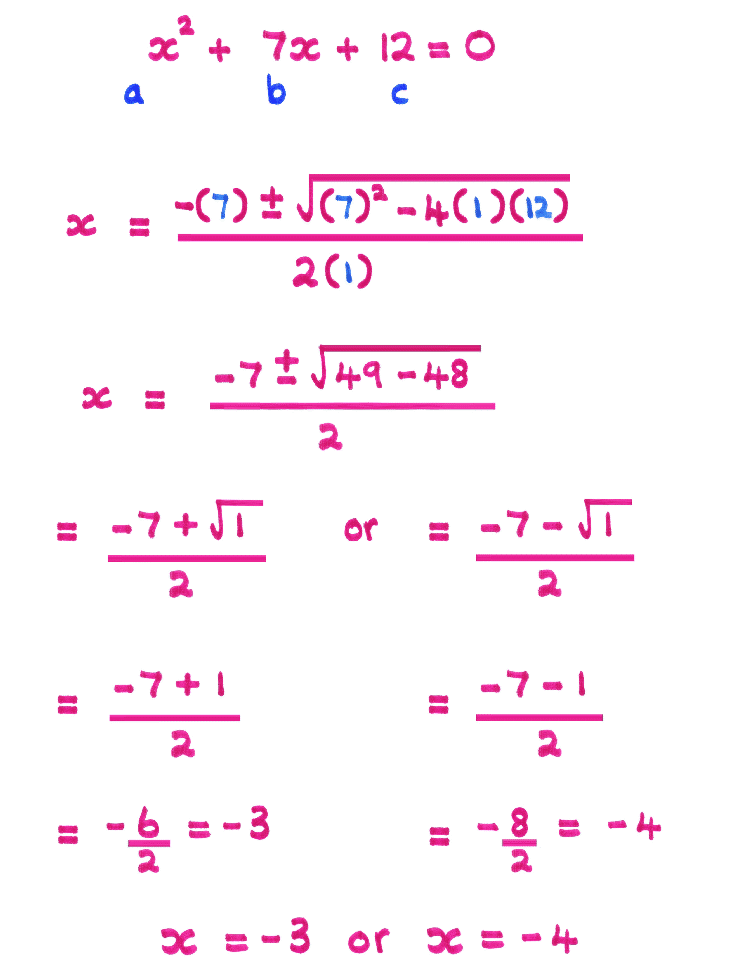Coming to Statistica
in 2022/2023

Zoom tutorials
using Explaineverything app
We a record sessions for you to listen to later

CALL STATISTICA NOW

.

E-mail     anne@statistica.com.au

# Place         Willetton, Western Australia# There are three algebraic ways of solving a quadratic equation

1. Factorising  two brackets multiplied equated to zero
2. Completing the Square equated to zero
3. Quadratic Formula gives you the solutions

Method One

You may have a common factor before you use quadratic factorising,
click Fug if you need help to remember the techniques.
If you have any amount of terms multiplied together to give the result zero
then one of the terms must be zero itself.

0    X      12    = 0
x
X   (x + 1) = 0  either x = 0 or (x + 1) = 0
x
X   (x + 1) (x  - 4)= 0  either x = 0  or  (x + 1) = 0 or   (x - 4) = 0Method Two

Use the formula for completing the square works no matter
what number is in front of the squared term
click Fug if you need help to remember the technique.

Remember the following:

1. Once in “ the completing the square ” form the next sign following
the bracket should be minus if the quadratic has real roots.
2. When you square root you will have a positive and a negative answer.
3. Use Algebra and take care with combining numbers and fractions here.

Click Fug for help with FractionsMethod Three

Use the quadratic formula and this works every time as some quadratics do
not factorise into brackets with whole numbers.
You may use the formula to find the whole numbers in the brackets by
reversing the sign of your solutions and putting them in the two brackets.

Click Fug if you need help to remember the technique.

Take care when substituting the values as remember negatives can prove to
be problems, particularly the value of b.
Put brackets around substituted values to help avoid problems.

Click Fug for help with Negatives[Home] [Parents] [Contact us] [Services] [Mathematics] [Statistics] [School]